# TOPIC4 Ultrasonic Waves PARAMETERS of wave Ultrasonic Waves

• Slides: 12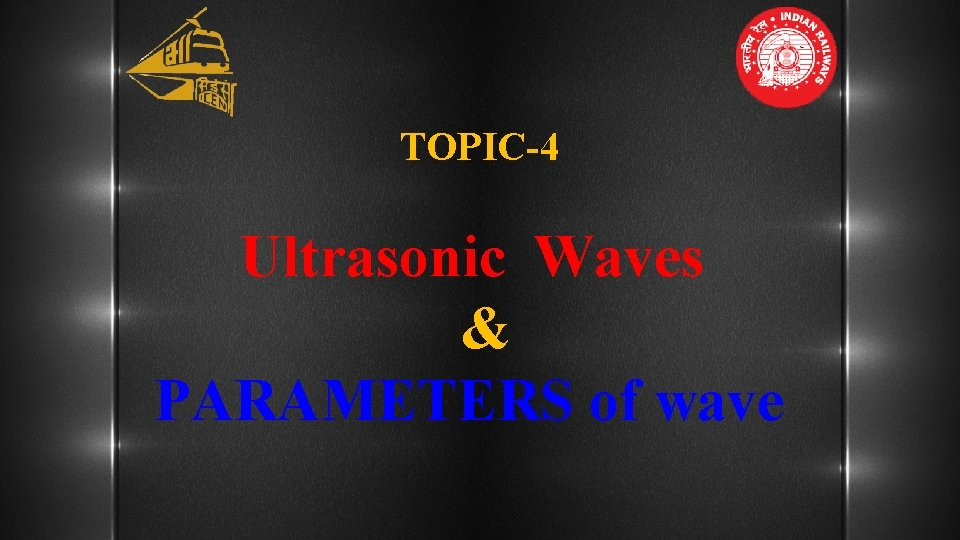TOPIC-4 Ultrasonic Waves & PARAMETERS of waveUltrasonic Waves § Ultrasonic waves are High frequency mechanical waves. § It Propagates in an elastic medium. § It Propagate in solid, liquid & gases due to the vibrations or oscillatory motion of particles within material. § Propagation in vacuum not possible. 21: 54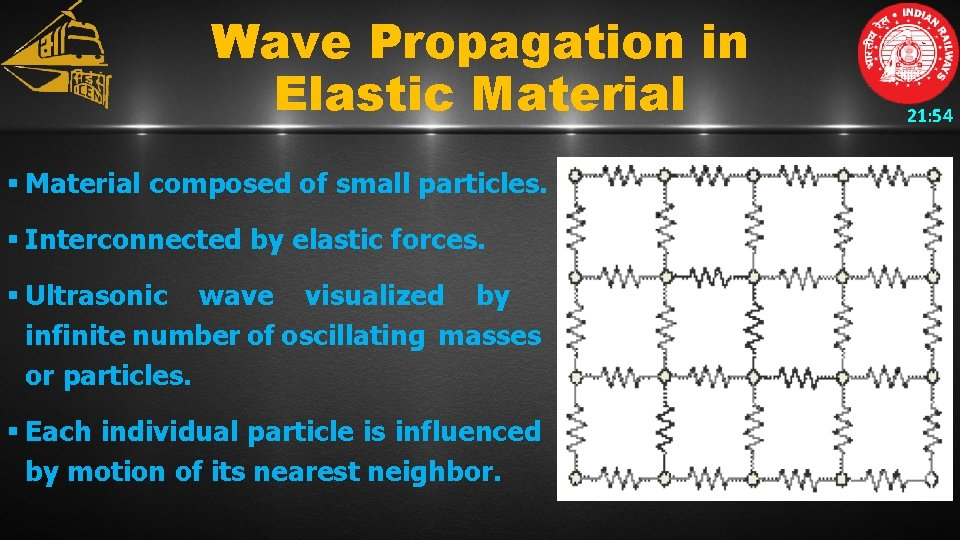Wave Propagation in Elastic Material § Material composed of small particles. § Interconnected by elastic forces. § Ultrasonic wave visualized by infinite number of oscillating masses or particles. § Each individual particle is influenced by motion of its nearest neighbor. 21: 54Wave Propagation in Elastic Material 21: 54 § Inertial and elastic restoring forces act upon each particle. § The forces tends to restore the particle to its original position. § The speed of propagation depends on elastic properties & density of the medium. § Different pattern of vibrational motion exist at atomic level. § Most of them irrelevant to Ultrasonic Testing.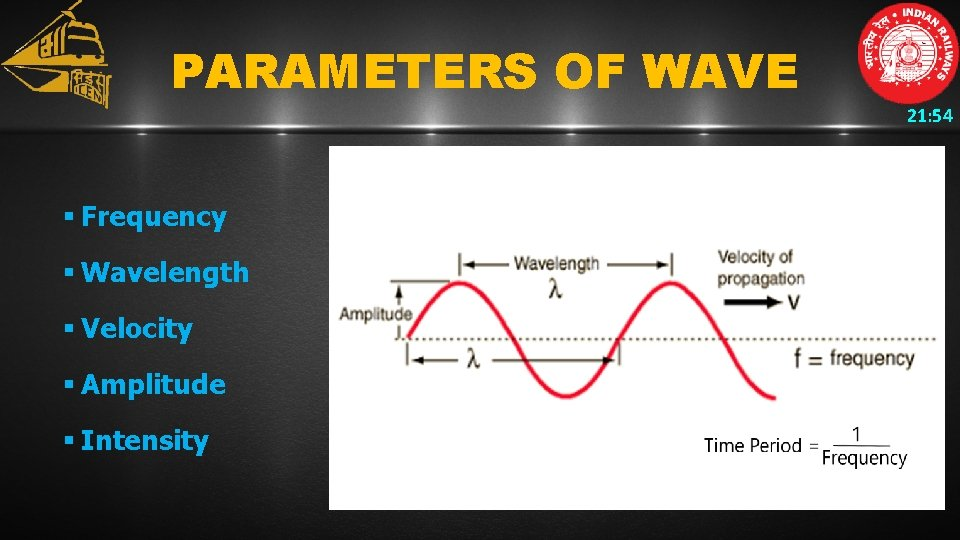PARAMETERS OF WAVE 21: 54 § Frequency § Wavelength § Velocity § Amplitude § IntensityFrequency 21: 54 § No. of oscillations/vibrations per second by a particle or object. § Oscillations is displacement from mean position of particle. § One Oscillation consist one crest & one trough § FREQUENCY is denoted by f § UNIT : Hz or Cycle/sec.Wavelength 21: 54 § Distance between Two consecutive planes allowing particle in the same state of motion i. e. § Distance between two consecutive (One after another) crest or trough. § Denoted by λ § UNIT: Meter (m)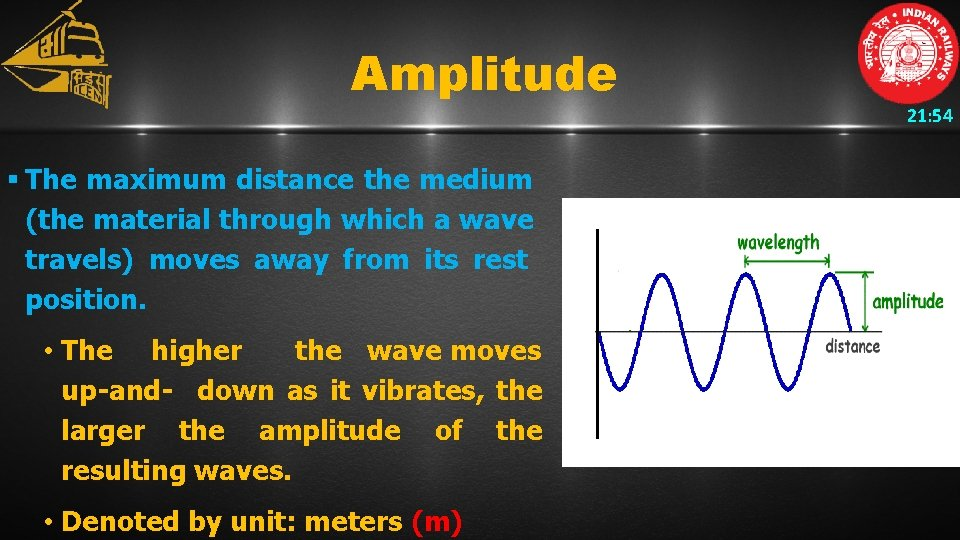Amplitude § The maximum distance the medium (the material through which a wave travels) moves away from its rest position. • The higher the wave moves up-and- down as it vibrates, the larger the amplitude of the resulting waves. • Denoted by unit: meters (m) 21: 54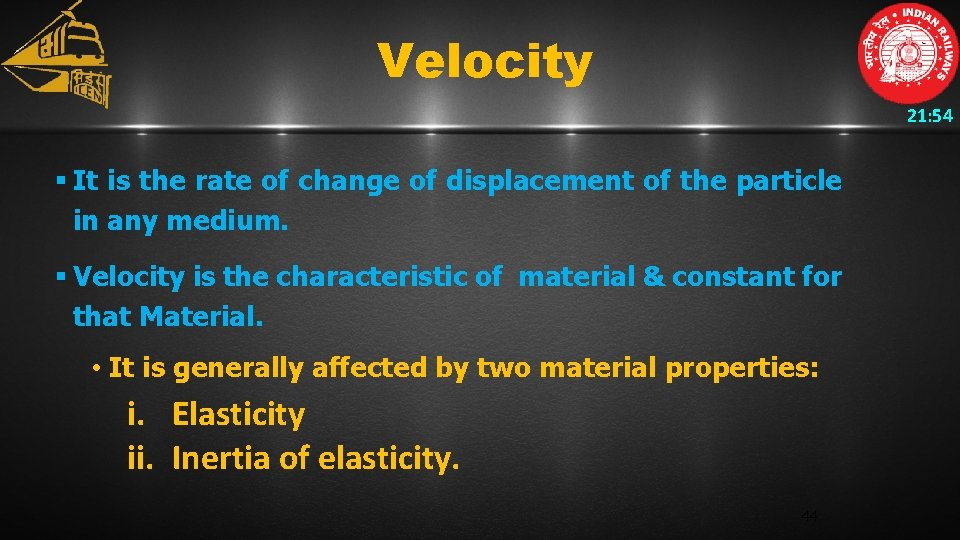Velocity 21: 54 § It is the rate of change of displacement of the particle in any medium. § Velocity is the characteristic of material & constant for that Material. • It is generally affected by two material properties: i. Elasticity ii. Inertia of elasticity. 44Velocity 21: 54 § Relation between velocity, frequency & wave length is Velocity (m/s) = Frequency (Hz) x Wavelength (m) V =f × λ Where, -V : Velocity in Km/sec or 103 m/s. -f : Frequency in Mc/s -λ : Wavelength in mm This Relation is valid for all type of waves.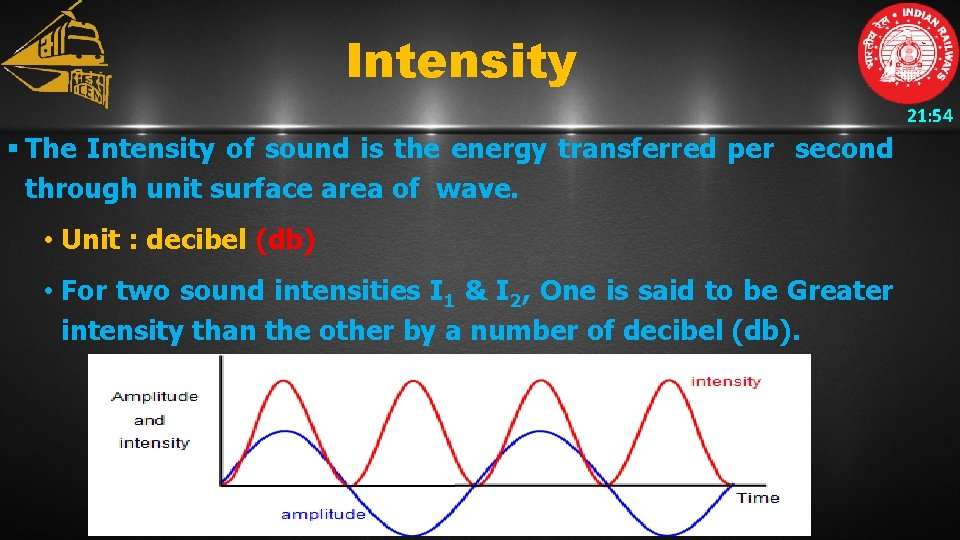Intensity 21: 54 § The Intensity of sound is the energy transferred per second through unit surface area of wave. • Unit : decibel (db) • For two sound intensities I 1 & I 2, One is said to be Greater intensity than the other by a number of decibel (db).Intensity 21: 54